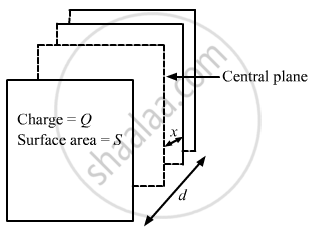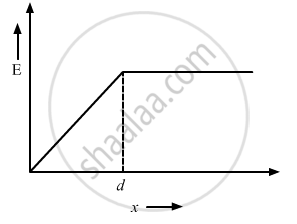Department of Pre-University Education, KarnatakaPUC Karnataka Science Class 12
Share

# A Non-conducting Sheet of Large Surface Area and Thickness D Contains a Uniform Charge Distribution of Density ρ . - Physics

ConceptElectric Field Introduction of Electric Field

#### Question

A non-conducting sheet of large surface area and thickness d contains a uniform charge distribution of density ρ. Find the electric field at a point P inside the plate, at a distance x from the central plane. Draw a qualitative graph of E against x for 0 < x < d.

#### Solution

Given:
Thickness of the sheet = d
Let the surface area of the sheet be s.
Volume of the sheet = sd
Volume charge density of the sheet, ρ = "Q"/"sd"
Charge on the sheet = QConsider an imaginary plane at a distance x from the central plane of surface area s.
Charge enclosed by this sheet, q =ρsx
For this Guassian surface, using Gauss's Law,we get:

oint  "E"."ds" = "q"/∈_0

"E"."s" = (ρ "sx")/∈_0

"E" =( ρ "x")/∈_0

The electric field outside the sheet will be constant and will be:

"E" =(ρ"d")/∈_0Is there an error in this question or solution?

#### APPEARS IN

Solution A Non-conducting Sheet of Large Surface Area and Thickness D Contains a Uniform Charge Distribution of Density ρ . Concept: Electric Field - Introduction of Electric Field.
S